# Difference between revisions of "Structured Types"

## Modelling Structured Types

The Event-B mathematical language currently does not support a syntax for the direct definition of structured types such as records or class structures. Nevertheless it is possible to model structured types using projection functions to represent the fields/attributes. For example, suppose we wish to model a record structure R with fields e and f (with type E and F respectively). Let us use the following 'invented' syntax for this (based on VDM syntax but currently not part of Event-B syntax):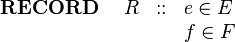$\begin{array}{lcl} \textbf{RECORD}~~~~ R &::& e\in E\\ && f \in F \end{array}$

We can model this structure in Event-B by introducing (in a context) a carrier set R and two functions e and f as constants as follows: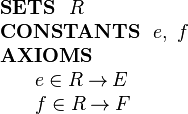$\begin{array}{l} \textbf{SETS}~~ R\\ \textbf{CONSTANTS}~~ e,~ f\\ \textbf{AXIOMS}\\ ~~~~\begin{array}{l} e \in R \tfun E\\ f \in R \tfun F\\ \end{array} \end{array}$

The (constant) functions e and f are projection functions that can be used to extract the appropriate field values. That is, given an element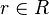$r\in R$ representing a record structure, we write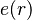$e(r)$ for the e component of r and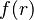$f(r)$ for the f component of r.

E and F can be any type definable in Event-B, including a type representing a record structure.

The approach to modelling structured types is based on a proposal by Evans and Butler . We refer to this approach to modelling records as a projection-based approach. Later we will look at a constructor-based approach and make the link between the two approaches.

## Constructing Structured Values

Suppose we have a variable v in a machine whose type is the structure R defined above: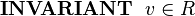$\textbf{INVARIANT}~~ v\in R$

We wish to assign a structured value to v whose e field has some value e1 and whose f field has some value f1. This can be achieved by specifying the choice of an event parameter r whose fields are constrained by appropriate guards and assigning parameter r to the machine variable v. This is shown in the following event: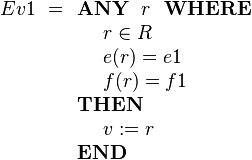$Ev1 ~=\begin{array}[t]{l} \textbf{ANY}~~ r ~~\textbf{WHERE} \\ ~~~~ r \in R \\ ~~~~ e( r ) = e1 \\ ~~~~ f( r ) = f1 \\ \textbf{THEN} \\ ~~~~ v := r \\ \textbf{END} \end{array}$

If we only wish to update some fields and leave others changed, this needs to be done by specifying explicitly that some fields remain unchanged. This is shown in the following example where only the e field is modified: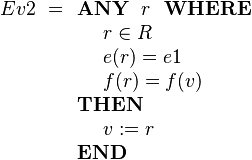$Ev2 ~=\begin{array}[t]{l} \textbf{ANY}~~ r ~~\textbf{WHERE} \\ ~~~~ r \in R \\ ~~~~ e( r ) = e1 \\ ~~~~ f( r ) = f(v) \\ \textbf{THEN} \\ ~~~~ v := r \\ \textbf{END} \end{array}$

If we don't care about the value of some field (e.g., f), we simply omit any guard on that field as follows: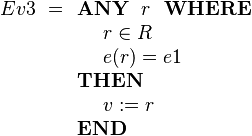$Ev3 ~= \begin{array}[t]{l} \textbf{ANY}~~ r ~~\textbf{WHERE} \\ ~~~~ r \in R \\ ~~~~ e( r ) = e1 \\ \textbf{THEN} \\ ~~~~ v := r \\ \textbf{END} \end{array}$

Sometimes we will wish to model a set of structured elements as a machine variable, e.g.,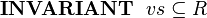$\textbf{INVARIANT}~~ vs\subseteq R$

We can add a structured element to this set using the following event: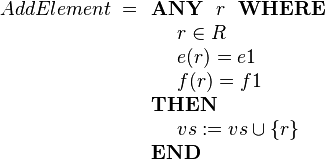$AddElement ~= \begin{array}[t]{l} \textbf{ANY}~~ r ~~\textbf{WHERE} \\ ~~~~ r \in R \\ ~~~~ e( r ) = e1 \\ ~~~~ f( r ) = f1 \\ \textbf{THEN} \\ ~~~~ vs := vs \cup \{r\} \\ \textbf{END} \end{array}$

## Extending Structured Types

In a refinement we can introduce new fields to an existing structured type. Suppose R is defined in context C1 with fields e and f as before. For the refinement, suppose we wish to add a field g of type G to structured type R. Let us use the following invented syntax for this (not part of Event-B syntax):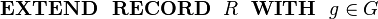$\begin{array}{l} \textbf{EXTEND~~ RECORD}~~ R ~~\textbf{WITH}~~ g\in G \end{array}$

This can be done by including the new field g as projection function on R in a context C2 (that extends C1). The new field is defined as follows: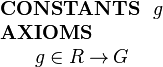$\begin{array}{l} \textbf{CONSTANTS}~~ g \\ \textbf{AXIOMS}\\ ~~~~\begin{array}{l} g \in R \tfun G \\ \end{array} \end{array}$

Above, we saw the AddElement event that adds a structured element to the set vs. We may want to specify a value for the new g field in the refinement of this event. Using the event extension mechanism, this can be achieved as follows: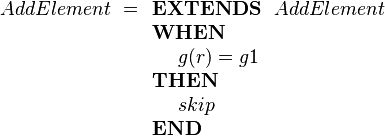$AddElement~= \begin{array}[t]{l} \textbf{EXTENDS}~~ AddElement \\ \textbf{WHEN} \\ ~~~~ g( r ) = g1 \\ \textbf{THEN} \\ ~~~~ skip \\ \textbf{END} \end{array}$

This is a form of superposition refinement where we add more data structure to the state by adding a new field to elements of the structured type R. In the above event we strengthen the specification by adding a guard using the event extension mechanism. The event extension mechanism means that this short form is the same as specifying the refined event as follows: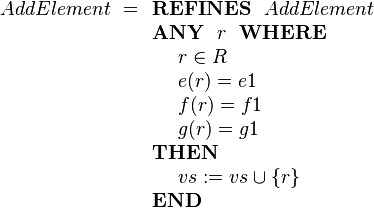$AddElement ~= \begin{array}[t]{l} \textbf{REFINES}~~ AddElement \\ \textbf{ANY}~~ r ~~\textbf{WHERE} \\ ~~~~ r \in R \\ ~~~~ e( r ) = e1 \\ ~~~~ f( r ) = f1 \\ ~~~~ g( r ) = g1 \\ \textbf{THEN} \\ ~~~~ vs := vs \cup \{r\} \\ \textbf{END} \end{array}$

## Sub-Typing

Extension, as described above, is used when adding new fields to a structured type as part of a refinement step. That is, at different abstraction levels, we may have different numbers of fields in a structured type.

Sometimes we wish to have different subtypes of a structure at the same abstraction level. We can achieve this by defining subsets of the structure type and defining projection functions for those subsets only. For example suppose we define a message type that has sender and receiver fields as well as a message identifier. In our invented notation this would be: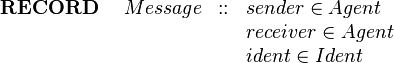$\begin{array}{lcl} \textbf{RECORD}~~~~ Message &::& sender\in Agent\\ && receiver \in Agent \\ && ident \in Ident \end{array}$

In Event-B this would be: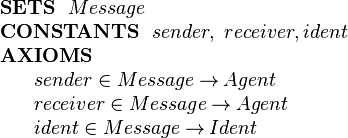$\begin{array}{l} \textbf{SETS}~~ Message\\ \textbf{CONSTANTS}~~ sender,~ receiver, ident\\ \textbf{AXIOMS}\\ ~~~~\begin{array}{l} sender \in Message \tfun Agent\\ receiver \in Message \tfun Agent\\ ident \in Message \tfun Ident \end{array} \end{array}$

We wish to have two subtypes of message, request messages and confirmation messages. We define two subsets of Message as follows: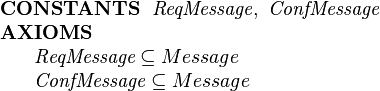$\begin{array}{l} \textbf{CONSTANTS}~~ \textit{ReqMessage},~ \textit{ConfMessage}\\ \textbf{AXIOMS}\\ ~~~~\begin{array}{l} \textit{ReqMessage} \subseteq Message \\ \textit{ConfMessage} \subseteq Message \\ \end{array} \end{array}$

We require requests to have a product and an amount field and a confirmation to have a price field. In our invented notation this would be specified as follows: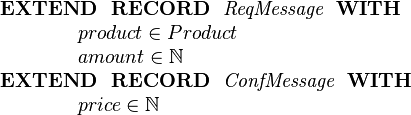$\begin{array}{l} \textbf{EXTEND~~ RECORD}~~ \textit{ReqMessage}~~\textbf{WITH}\\ ~~~~~~~~~~~~ product\in Product\\ ~~~~~~~~~~~~ amount\in \nat\\ \textbf{EXTEND~~ RECORD}~~ \textit{ConfMessage}~~\textbf{WITH}\\ ~~~~~~~~~~~~ price\in \nat\\ \end{array}$

In Event-B the additional fields are specified as projection functions on the relevant message subsets: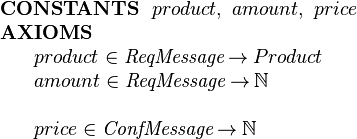$\begin{array}{l} \textbf{CONSTANTS}~~ product,~ amount,~ price\\ \textbf{AXIOMS}\\ ~~~~\begin{array}{l} product \in \textit{ReqMessage} \tfun Product\\ amount \in \textit{ReqMessage} \tfun \nat\\ ~\\ price \in \textit{ConfMessage} \tfun \nat \end{array} \end{array}$

Typically we would require that the message subtypes are disjoint. In that case we would add an additional axiom: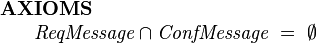$\begin{array}{l} \textbf{AXIOMS}\\ ~~~~\begin{array}{l} \textit{ReqMessage} \cap \textit{ConfMessage} ~=~ \emptyset \\ \end{array} \end{array}$

If we know that there are no other subtypes besides requests and confirmations and that they should be disjoint, then we can condense the subset and disjointness axioms into a partitions axiom: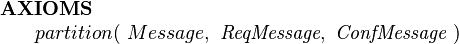$\begin{array}{l} \textbf{AXIOMS}\\ ~~~~\begin{array}{l} partition(~ Message, ~\textit{ReqMessage},~ \textit{ConfMessage} ~) \end{array} \end{array}$

Suppose we have 2 variables representing request and confirmation channels repsectively: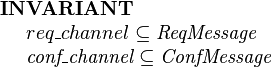$\begin{array}{l} \textbf{INVARIANT}~~ \\ ~~~~req\_channel \subseteq \textit{ReqMessage} \\ ~~~~\textit{conf\_channel} \subseteq \textit{ConfMessage} \end{array}$

The following Confirm event models an agent responding to request by issuing a confirmation message. The Confirm event is enabled for the agent if there is a request message, req, whose receiver is that agent. The effect of the event is to chose a confirmation message, conf, whose fields are constrained with appropriate guards and add that confirmation message to the confirmations channel: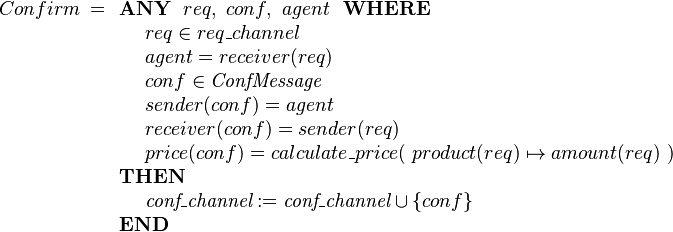$Confirm ~= \begin{array}[t]{l} \textbf{ANY}~~ req,~ conf, ~ agent ~~\textbf{WHERE} \\ ~~~~ req \in req\_channel \\ ~~~~ agent = receiver(req) \\ ~~~~ conf \in \textit{ConfMessage} \\ ~~~~ sender( conf ) = agent \\ ~~~~ receiver( conf ) = sender(req) \\ ~~~~ price(conf) = calculate\_price(~ product(req)\mapsto amount(req) ~) \\ \textbf{THEN} \\ ~~~~ \textit{conf\_channel} := \textit{conf\_channel} \cup \{conf\} \\ \textbf{END} \end{array}$

It might seem that we should always specify that subtypes are disjoint, but this is not so. Not making them disjoint allows us to represent a form of 'mulitple inheritance'. For example, we could declare a new subtype ReqConfMessage that subsets both ReqMessage and ConfMessage: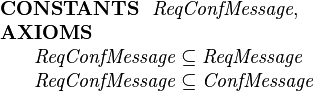$\begin{array}{l} \textbf{CONSTANTS}~~ \textit{ReqConfMessage},\\ \textbf{AXIOMS}\\ ~~~~\begin{array}{l} \textit{ReqConfMessage} \subseteq \textit{ReqMessage} \\ \textit{ReqConfMessage} \subseteq \textit{ConfMessage} \\ \end{array} \end{array}$

Elements of ReqConfMessage will have the fields of both subtypes, i.e., sender, receiver, ident, product, amount and price.

This combined subtype could be further extended: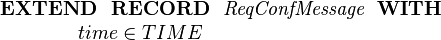$\begin{array}{l} \textbf{EXTEND~~ RECORD}~~ \textit{ReqConfMessage}~~\textbf{WITH}\\ ~~~~~~~~~~~~ time\in TIME\\ \end{array}$

For an example of a Rodin development that uses the projection-based approach to modelling and extending records see 

## Constructor-based Records

An alternative style of modelling records is to define constructor functions that construct a record from a tuple of the components of that record. This is the approach that VDM follows, for example. Suppose we wish to model the following record structure:$\begin{array}{lcl} \textbf{RECORD}~~~~ R &::& e\in E\\ && f \in F \end{array}$

We introduce the constructor function for R records, mk-R, as follows: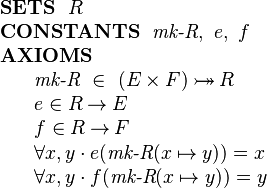$\begin{array}{l} \textbf{SETS}~~ R\\ \textbf{CONSTANTS}~~ \textit{mk-R},~ e,~ f\\ \textbf{AXIOMS}\\ ~~~~\begin{array}{l} \textit{mk-R} ~\in~ (E\times F) \tbij R\\ e \in R \tfun E\\ f \in R \tfun F\\ \forall x,y \cdot e( \textit{mk-R}(x\mapsto y) ) = x \\ \forall x,y \cdot f( \textit{mk-R}(x\mapsto y) ) = y \end{array} \end{array}$

The mk-R function is specified as a constructor for type R that takes an element of type E and an element of type F and constructs an value of type R. The constructor is specified as injective because when the components are different, then the corresponding records should be different. The above declaration also makes the constructor surjective which means that the constructor provides a way of generating all records of type R.

With the constructor based approach, the AddElement event already shown above does not require a selection of a record satisfying the required properties. Instead we use the constructor directly: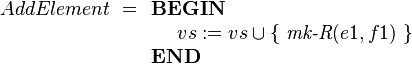$AddElement ~= \begin{array}[t]{l} \textbf{BEGIN}\\ ~~~~ vs := vs \cup \{~ \textit{mk-R}(e1,f1) ~\} \\ \textbf{END} \end{array}$

It is possible to define different subtypes in a similar way as in the projection-based approach by defining separate constructors for each (disjoint) subtype.

Adding new fields in a refinement is more difficult with the constructor approach however. We would need to introduce a completely new constructor for the full extended record structure and to get the refinement to work correctly, we would need to define a relationship between the original record and the extended version. This merits further exploration.

## Projections versus Constructors

As we have seen, the constructor approach can lead to more succinct event specifications than the projection approach. The downside of the constructor approach is that it is difficult to extend records in refinement steps and stepwise refinement and introduction of structure through refinement is an major feature of the Event-B approach.

We could say that the projection approach gives us open record structures, that is, structures that are easy to extend in a refinement. The constructor approach gives us closed records that are not directly extendable in refinement steps.

The constructor approach can be seen as a strengtening of the projection approach as is now explained. Consider again the two projection functions introduced to represent fields of the record structure R in the projection approach: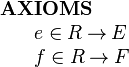$\begin{array}{l} \textbf{AXIOMS}\\ ~~~~\begin{array}{l} e \in R \tfun E\\ f \in R \tfun F \end{array} \end{array}$

The direct product of these two projections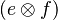$(e \otimes f)$ has the following type: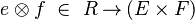$\begin{array}{l} ~~~~\begin{array}{l} e \otimes f ~\in~ R \tfun (E\times F) \end{array} \end{array}$

The inverse of the direct product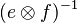$(e \otimes f)^{-1}$ relates pairs of type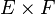$E\times F$ to elements of type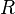$R$. If we specify that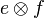$e \otimes f$ is injective, i.e., its inverse is a function, then$(e \otimes f)^{-1}$ becomes a constructor function for records of type$R$.

This means that an open record structure can be 'closed-off' by the addition of an axiom stating that the direct product of the fields is injective: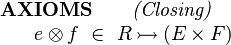$\begin{array}{l} \textbf{AXIOMS}~~~~~~\textit{ (Closing) }\\ ~~~~\begin{array}{l} e \otimes f ~\in~ R \tinj (E\times F) \end{array} \end{array}$

Once this closing axiom is introduced, we can no longer introduce new fields to the record structure - at least not without introducing an inconsistency. If we were to now add a field g, the closing axiom would mean that records whose e and f fields are the same, must be correspond to the same record r even if they differ in the g field. But since g is a function, different g values cannot be related to the same record r.

Regardless of whether the closing axiom is introduced, some feasibility proof obligations arising from the use of the projection approach will require the existence of a record corresponding to any tuple of field components. This can be specified by the following feasibility axiom stating that the product of the projections is surjective: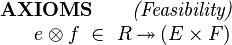$\begin{array}{l} \textbf{AXIOMS}~~~~~~\textit{ (Feasibility) }\\ ~~~~\begin{array}{l} e \otimes f ~\in~ R \tsur (E\times F) \end{array} \end{array}$

## Recursive Structured Types

It is well known how to deal with recursive records using the constructor approach. This is explored further in the context of Event-B and Rodin as part of the mathematical extension work where proposals for inductive datatypes are made (see Mathematical_extensions). Inductive datatypes are defined by constructor functions similar to constructor-based records. For example, to define lists we would use two disjoint constructors, nil and cons. The cons constructor for lists typically comes with two projections, head and tail. The structural induction principle for inductive datatypes is well known.

With the projection approach we can define two subtypes, for empty and non-empty lists, and extend the non-empty subtype with head and tail projections.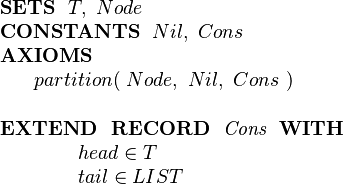$\begin{array}{l} \textbf{SETS}~~ T,~ Node\\ \textbf{CONSTANTS}~~ Nil,~ Cons\\ \textbf{AXIOMS}\\ ~~~~\begin{array}{l} partition(~ Node, ~Nil,~ Cons ~) \end{array} \\~\\ \textbf{EXTEND~~ RECORD}~~ \textit{Cons}~~\textbf{WITH}\\ ~~~~~~~~~~~~ head\in T \\ ~~~~~~~~~~~~ tail\in LIST \end{array}$

The appropriate induction principle for the projection based approach needs to be explored further.

To be done...